# (1 point) Consider the Initial Value Problem xi(0) 6 = 10xi-4x2 (a) Find the eigenvalues and eige...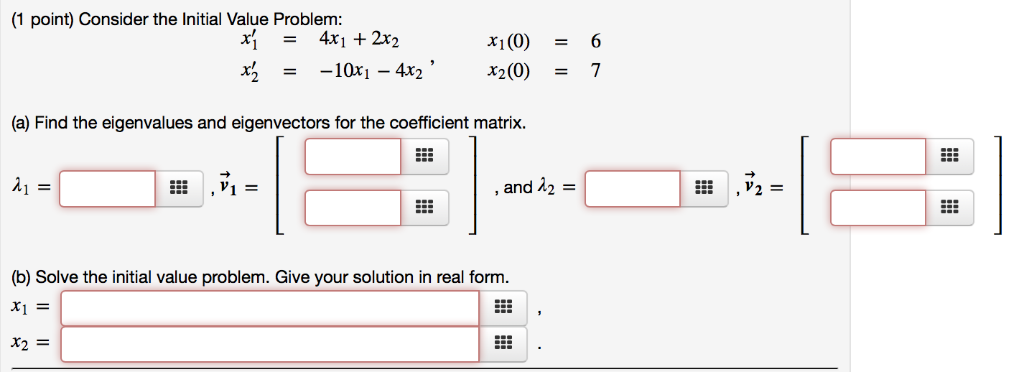(1 point) Consider the Initial Value Problem xi(0) 6 = 10xi-4x2 (a) Find the eigenvalues and eigenvectors for the coefficient matrix. ,V2- and 12 (b) Solve the initial value problem. Give your solution in real form x1F X2=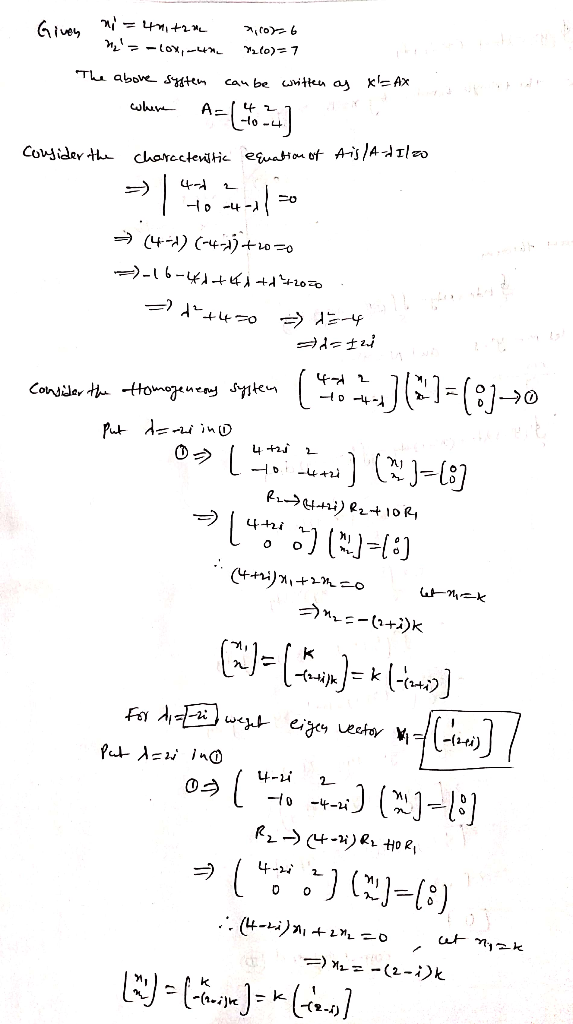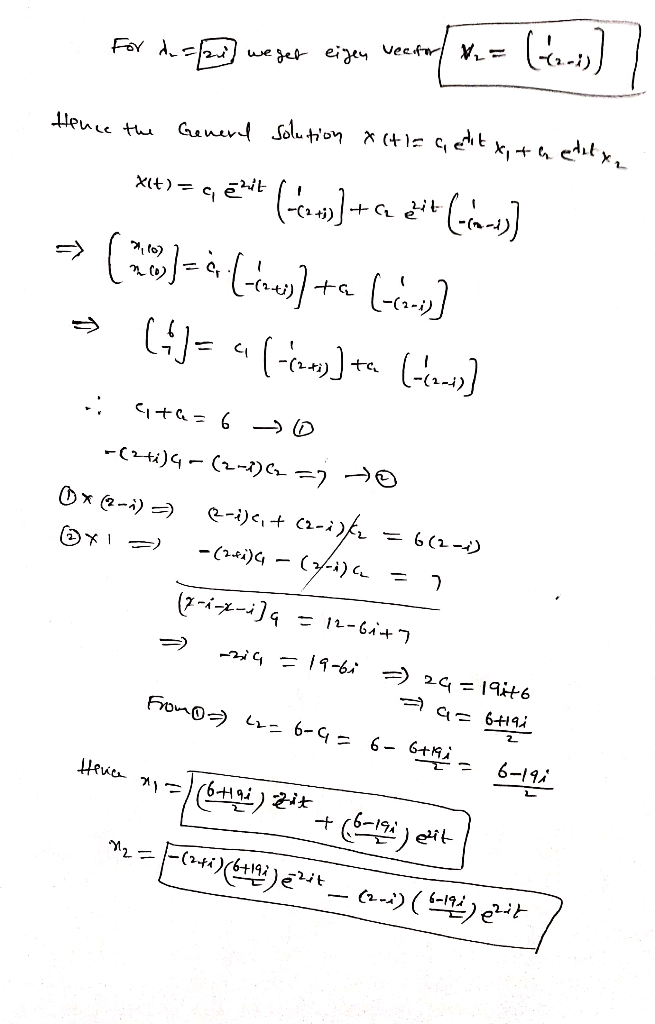#### Earn Coin

Coins can be redeemed for fabulous gifts.

Similar Homework Help Questions
• ### (1 point) Consider the initial value problem (a) Find the eigenvalues and eigenvectors for the coefficient...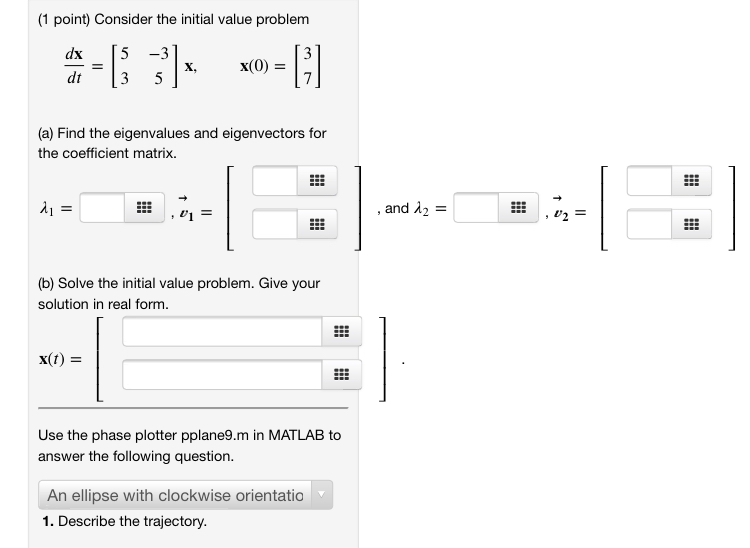(1 point) Consider the initial value problem (a) Find the eigenvalues and eigenvectors for the coefficient matrix. di = , and 12 = (b) Solve the initial value problem. Give your solution in real form. X(t) = Use the phase plotter pplane9.m in MATLAB to answer the following question. An ellipse with clockwise orientatio 1. Describe the trajectory.

• ### (1 point) Consider the initial value problem (a) Find the eigenvalues and eigenvectors for the coefficient...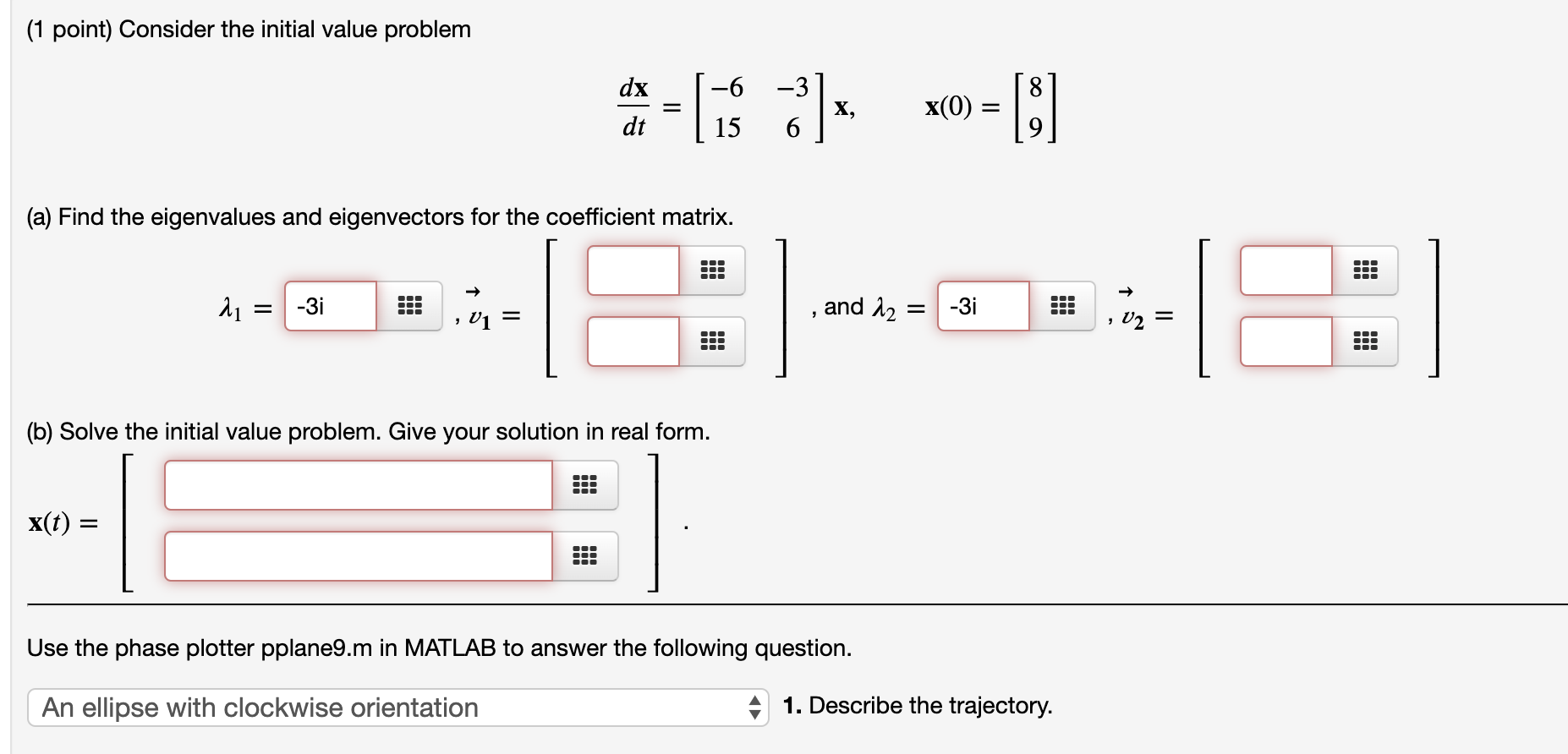(1 point) Consider the initial value problem (a) Find the eigenvalues and eigenvectors for the coefficient matrix. 11 = -3i .. , , and 12 = -3i , 01 (b) Solve the initial value problem. Give your solution in real form. X(t) = Use the phase plotter pplane9.m in MATLAB to answer the following question. An ellipse with clockwise orientation 41. Describe the trajectory.

• ### (1 point) Consider the Initial Value Problem -5 dx dt X x(0) (a) Find the eigenvalues...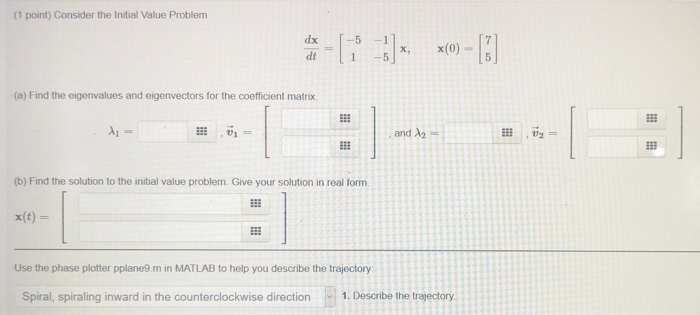(1 point) Consider the Initial Value Problem -5 dx dt X x(0) (a) Find the eigenvalues and eigenvectors for the coefficient matrix A = and 2 -- 1 333 (b) Find the solution to the initial value problem. Give your solution in real form Use the phase plotter pplane9.m in MATLAB to help you describe the trajectory Spiral, spiraling inward in the counterclockwise direction 1. Describe the trajectory

• ### (1 point) Consider the Initial Value Problem 7-177] - (a) Find the eigenvalues and eigenvectors for...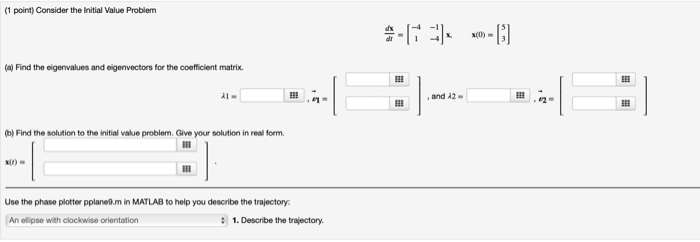(1 point) Consider the Initial Value Problem 7-177] - (a) Find the eigenvalues and eigenvectors for the coefficient matrix. Find the solution to the initial value problem. Give your solution in real form. Use the phase plotter pplanom in MATLAB to help you describe the trajectory An ellipse with clockwise orientation • 1. Describe the trajectory.

• ### 7.6(3) (1 point) Consider the Initial Value Problem -L* 4)*, x0=[!] (a) Find the eigenvalues and...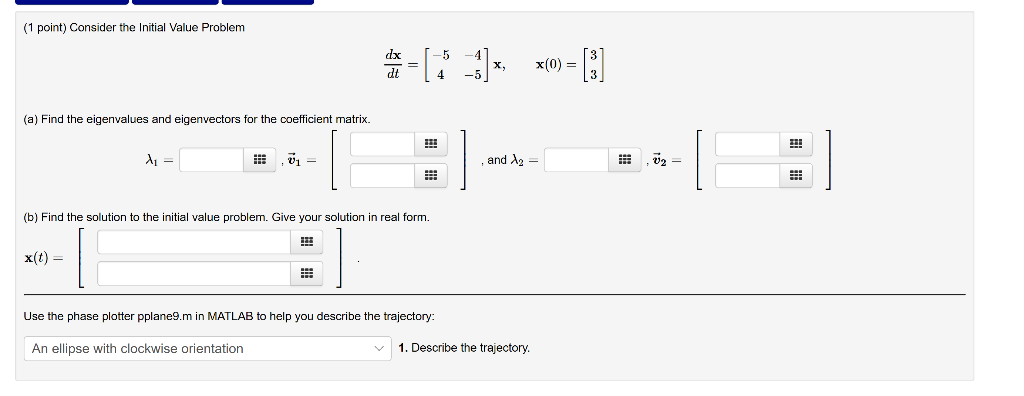7.6(3) (1 point) Consider the Initial Value Problem -L* 4)*, x0=[!] (a) Find the eigenvalues and eigenvectors for the coefficient matrix. 1 ,01 = , and 12 = (b) Find the solution to the initial value problem. Give your solution in real form. x(t) = Use the phase plotter pplane9.m in MATLAB to help you describe the trajectory An ellipse with clockwise orientation 1. Describe the trajectory.

• ### (1 point) Consider the Initial Value Problem: = 's = -9x1 + 3x2 -3021 + 9x2...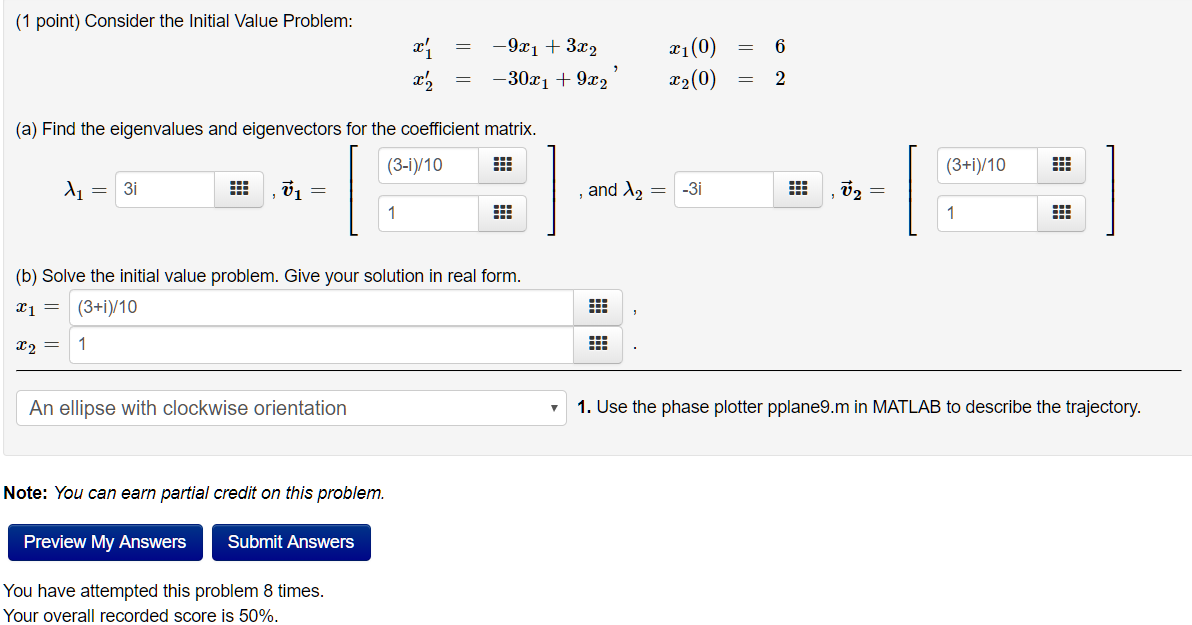(1 point) Consider the Initial Value Problem: = 's = -9x1 + 3x2 -3021 + 9x2 xi(0) = 6 22(0) = 2 (a) Find the eigenvalues and eigenvectors for the coefficient matrix. (3-1)/10 (3+1)/10 l1 = 3i , = , and 12 = -3i , ū2 = | (b) Solve the initial value problem. Give your solution in real form. x1 = (3+1)/10 x2 = 1 An ellipse with clockwise orientation 1. Use the phase plotter pplane9.m in MATLAB to...

• ### Problem 5. (1 point) Consider the linear system a. Find the eigenvalues and eigenvectors for the...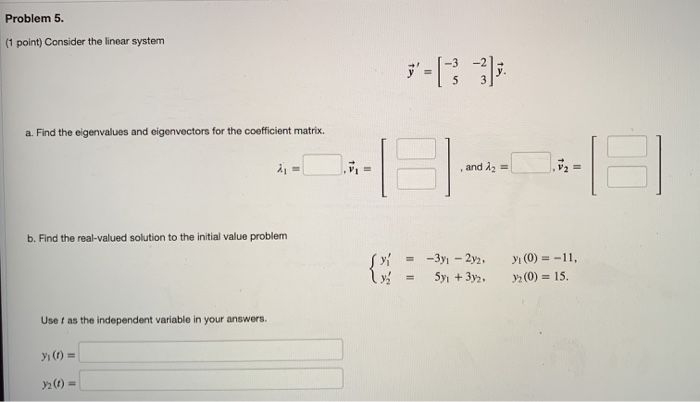Problem 5. (1 point) Consider the linear system a. Find the eigenvalues and eigenvectors for the coefficient matrix. 1 = . and 12 = V2 = b. Find the real-valued solution to the initial value problem = -3y - 2y, 5y + 3y2 (0) = -11, y (0) = 15. Usef as the independent variable in your answers. y (t) = (1) =

• ### Section 7.6 Complex Eigenvalues: Problem 5 Previous Problem Problem List Next Problem (1 point) Consider the...Section 7.6 Complex Eigenvalues: Problem 5 Previous Problem Problem List Next Problem (1 point) Consider the initial value problem date [10 ] x x(0) =  (a) Find the eigenvalues and eigenvectors for the coefficient matrix. X = * , ū = (b) Solve the initial value problem. Give your solution in real form. x(t) = Use the phase plotter pplane9.m in MATLAB to answer the following question An ellipse with clockwise orientation 1. Describe the trajectory

• ### Problem 5. (1 point) Consider the linear system a. Find the eigenvalues and eigenvectors for the...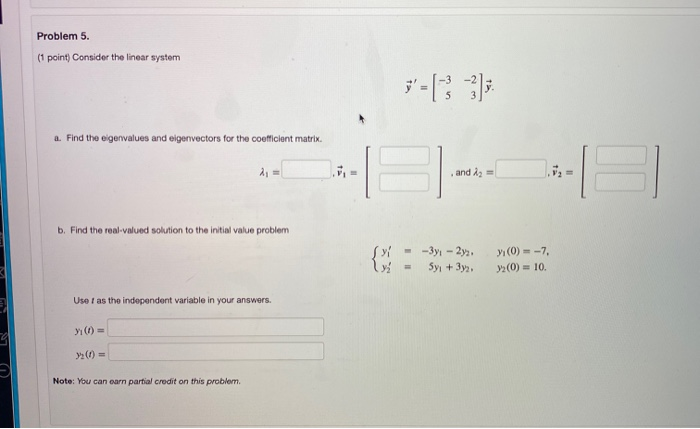Problem 5. (1 point) Consider the linear system a. Find the eigenvalues and eigenvectors for the coefficient matrix. and iz = b. Find the real-valued solution to the initial value problem - -3y - 2y2 Syı + 3y2 yı(0) = -7, (0) = 10 Use I as the independent variable in your answers. Y() = Note: You can earn partial credit on this problem. Problem 6. (1 point) Find the most general real-valued solution to the linear system of differential...

• ### (1 point) Consider the initial value problem dx [2 -5 dt 15 2 (a) Find the...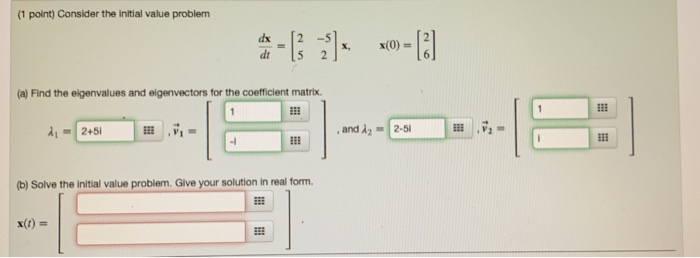(1 point) Consider the initial value problem dx [2 -5 dt 15 2 (a) Find the eigenvalues and elgenvectors for the coefficient matrix and λ2-2-51 (b) Solve the initial value problem. Give your solution in real fornm. x(t)